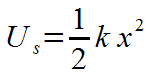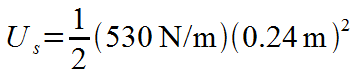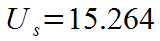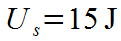### Calculate spring potential energy given the spring constant and the spring extension.

#### Example question:

How much potential energy will a linear spring have if it has a constant of 530 N/m and a displacement stretch of 0.24 m?

#### Given:

spring constant: k = 530 N/m

displacement: x = 0.24 mStarting formulaPlug in numbers with unitsMultiply: (1/2)(530)(0.24^2) = 15.264Final result with significant figures and unit